# Multi-junction electrical solver¶

﻿A complete photovoltaic solar cell can include one or more junctions, metal contacts, optical layers (including anti-reflective coatings and nano-photonic structures) and tunnel junctions. The junctions, in turn, might range from simple PN homojunctions to complex heterojunctions, including multi-quantum well structures. The solvers described so far only calculate the properties of single junction devices. To combine them into a multi-junction device, it is necessary to consider that the individual junctions are electrically connected in series and the potential coupling of light emitted by the wider bandgap junctions into those with smaller bandgap.

We first consider the case of no radiative coupling between junctions. This is a good approximation for materials which do not radiate efficiently or radiative materials working at low concentration, when the fraction of radiative recombination compared to non-radiative recombination is low. In this case, the IV curve of each junction can be calculated independently of each other and the current flowing through the MJ structure is limited by the junction with the lowest current at any given voltage. Series resistances defined for each junction are now added together and included as a single term. The operating voltage of each of the junctions is finally back-calculated and added together to get the voltage of the MJ device.

The pseudocode for this solver is:

1. Calculate the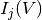of each junction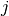in the structure.
2. Find the current flowing through the MJ device as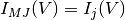, if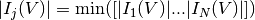.
3. Calculate the voltage of each junction by interpolating its IV curve at the new current values,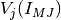, and the voltage dropped due to the series resistances,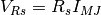.
4. Calculate the total voltage at a given current as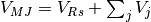.
5. Interpolate the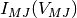and the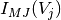to the desired output voltage values.

The figure shows the simulated IV curve of a 3J solar cell made of Ge/InGaAs/GaAsP. The electrical properties of the three junctions were calculated using the depletion approximation solver. In the dark the voltages of each of the junctions at a given current add together, resulting in the total voltage of the MJ structure. The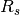contribution to the voltage goes in the same direction as those of the junctions. Under illumination the junction producing the lower current (the top junction in this case) limits the overall current of the MJ device. At zero bias, or even at some negative bias, the non-limiting junctions are positively biased, recombining all the photocurrent that cannot be extracted because of the limiting top cell. The contribution of theto the voltage of the MJ device is negative, resulting in a reduction of the fill factor and the overall efficiency of the solar cell.Radiative coupling takes place when the light produced by a high bandgap junction due to radiative recombination is absorbed by a lower bandgap junction, contributing to its photocurrent and changing the operating point. It has been identified in numerous highly radiative materials such as quantum well solar cells and III-V MJ solar cells . It appears as an artefact during the QE measurements of MJ solar cells but it is also an effect that can be exploited to increase the performance of MJ devices and their tolerance to spectral changes, resulting in superior annual energy yield.

The radiative coupling formalism included in Solcore is based on the works by Chan et al. (2014) and Nelson et al. (1997).

For a more detailed description of the radiative coupling calculator refer to the main Solcore paper (open access) and references therein.

An example of the radiative coupling in action can be found here.

## Tunnel junctions¶

Solcore includes partial support for tunnel junctions. They represent an optical loss due to parasitic absorption in the layers, but also an electrical loss. At the moment, there are two models for tunnel junctions. The first one is a simple resistive model, where the tunnel junction is simply modelled as a series resistance. This approximation should be valid in most cases, but will break down if the current is close to or higher than the peak current density of the junction.

The second model is a parametric model, based on the simple formalism described by Sze. In this model, the total current of the tunnel junction will have three components: the tunnel current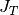accounting for band-to-band transport, the excess current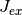related to transport across states inside the forbidden gap, and the diffusion current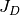, which is the usual minority-carrier injection current in PN junctions. The following equations summarise all these components.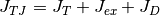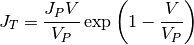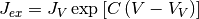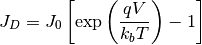As illustrated in the next figure,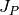and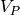are the peak current and voltage,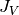and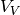are the valley current and voltages,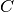is a prefactor of the exponent andthe reverse saturation current. In this simple implementation, these 6 parameters need to be provided as inputs, and can be used as fitting parameters to reproduce experimental data. This allows to correctly account for the break down of the tunnel junction in situations when the current is above the peak current. The code for this example can be found here.Solcore can also accept external IV data for the tunnel junctions and the implementation of the more rigorous, but still analytic model, described by Louarn et al. (2016) is currently under way in order to relate the tunnel currents with the actual materials and layer structure used in the tunnel junction definition.

## Multi-junction IV functions¶

solcore.analytic_solar_cells.IV.iv_multijunction(solar_cell, options)[source]

Calculates the overall IV characteristics of any number of junctions numerically at the requested voltage points. If photocurrent is not provided, the resultant IV characteristics are purely recombination currents, otherwise light IVs are returned.

In the end, the soalr_cell object is updated with an “iv” attribute containing a dictionary with:
“IV”: (V, I) Calculated IV characteristics “junction IV”: [(V junc 1, I junc 1), (V junc 2, I junc 2), …] “Rseries IV”: (V, I) Calculated IV characteristics of the series resistance “coupled IV”: For each junction but for the first one, a list with the coupled current coming form the upper junctions. “Isc”, Voc”, “P”, “FF” and “Eta”: In case of mpp = True and light IV.
The sign convention is:
• Photocurrents: Positive.
• Dark Currents: Negative
Parameters: solar_cell – A solar cell object with one or more junctions. The IV of the individual junctions must have been calculated already. kwargs – A dictionary containing, at least, the following elements: - mpp: (Boolean) If Isc, Voc, FF, Vmpp, Impp and Pmpp must be calculated. - voltages: Array of voltages in which to calculate the data - light_iv: (Boolean) if light IV is being calculated None
solcore.analytic_solar_cells.IV.solve_radiative_coupling(solar_cell, options, V_junction_array)[source]

Calculates the radiative IV curve of a MJ solar cell in the presence of radiative coupling between subcells.

WARNING: Tunnel junctions are not implemented in the radiative coupling mode, yet.

Parameters: solar_cell – The MJ solar cell structure options – General options of the solver V_junction_array – Array with all the junction voltages without coupling. Only the first element is used, actually. A tupple with J, V_junction_array in the presence of coupling and the coupled current
solcore.analytic_solar_cells.tunnel_junctions.resistive_tunnel_junction(junction, options)[source]

Calculates the IV curve of a tunnel junction when it is modelled as a simple resistor. The minimum resistance of the junction is always 1e-16.

Parameters: junction – A junction object. options – Solver options. None.
solcore.analytic_solar_cells.tunnel_junctions.parametric_tunnel_junction(junction, options)[source]

Calculates the IV curve of a tunnel junction when it is modelled using a set of empirical parameters, such as peak curent and voltage, valley current and voltage, etc. The total current of the tunnel junction is summ of 3 components: the tunnel current, the excess current and the difussion current.

Parameters: junction – A junction object. options – Solver options. None.
solcore.analytic_solar_cells.tunnel_junctions.external_tunnel_junction(junction, options)[source]

Calculates the IV curve of a tunnel junction when it is modelled with external data. The external voltage and current must be defined with the input parameters external_voltage and external_current, respectively. It assumes that the interesting part of the curve is in the 1st quadrant (V>0 and I>0).

Parameters: junction – A junction object. options – Solver options. None.
solcore.analytic_solar_cells.tunnel_junctions.example_resistive_tunnel_junction(show=True)[source]
solcore.analytic_solar_cells.tunnel_junctions.example_parametric_tunnel_junction(show=True)[source]